American Journal of Applied Mathematics
Volume 3, Issue 3, June 2015, Pages: 90-94

The Comparison Adomian Decomposition Method and Differential Quadrature Method for Solving Some Nonlinear Partial Diferential Equations

Department of Mathematics, Faculty of Mathematical Sciences, University of Mazandaran, Babolsar, Iran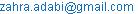Zahra Adabi Firoozjae, Allahbakhsh yazdani. The Comparison Adomian Decomposition Method and Differential Quadrature Method for Solving Some Nonlinear Partial Diferential Equations. American Journal of Applied Mathematics. Vol. 3, No. 3, 2015, pp. 90-94. doi: 10.11648/j.ajam.20150303.12

Abstract: Nonlinear partial diferential equations are a class of partial diferential equations having many important uses in engineering and sciences. In this work we display a comparison between Adomian Decomposition Method (ADM) and Differential Quadrature Method (DQM) for solving some nonlinear partial diferential equations. We found the existence of exact solutions for those models. The numerical results show the efficiency and accuracy of this method.

Contents

1. Introduction

Nonlinear partial differential equations can be found in wide variety scientific and engineering applications. Many important mathematical models can be expressed in terms of nonlinear partial differential equations. The most general form of nonlinear partial differential equation is given by:

F(u,ut ,ux ,u y , x, y,t) = 0                      (1a)

with initial and boundary conditions

u(x, y,0) =φ (x, y),x, yΩ,ΩR2               (1b)

u(x, y, t) = f (x, y,t),x, y∂Ω                  (1c)

where Ω is the solution region and ∂Ω is the boundary of Ω.

In recent years, much research has been focused on the numerical solution of nonlinear partial equations by using numerical methods and developing these methods [1,2]. In the numerical methods, which are commonly used for solving these kind of equations large size or difficult of computations is appeared and usually the round-off error causes the loss of accuracy. The Adomian decomposition method which needs less computation was employed to solve many problems [3,4]. Therefore, we applied the Adomian decomposition method to solve some models of nonlinear partial equation, this study reveals that the Adomian decomposition method is very efficient for nonlinear models, and it results give evidence that high accuracy can be achieved.

The Adomian decomposition method (ADM)  is a well-known systematic method for prac- tical solution of linear or nonlinear and deterministic or stochastic operator equations, including ordinary diferential equations (ODEs), partial diferential equations (PDEs), integral equations, integro-diferential equations, etc. The ADM is a powerful technique, which provides effcient- gorithms for analytic approximate solutions and numeric simulations for real-world applications in the applied sciences and engineering. It permits us to solve both nonlinear initial value problems(IVPs) and boundary value problems (BVPs) [6, 7] without unphysical restrictive assumptions such as required by linearization, perturbation, ad hoc assumptions, guessing the initial term or a set of basis functions, and so forth. Furthermore the ADM does not require the use of Green's functions, which would complicate such analytic calculations since Green's functions are not easily determined in most cases. The accuracy of the analytic approximate solutions obtained can be verifed by direct substitution. Advantages of the ADM over Picard's iterated method were demonstrated in . More advantages of the ADM over the variational iteration method were presented in [9, 10]. A key no- tion is the Adomian polynomials, which are tailored to the particular nonlinearity to solve nonlinear operator equations.

The principle of the Adomian decomposition method (ADM) when applied to a general nonlinear equation is in the following form (11):

Lu + Ru + Nu = g                          (2)

inverse operator L , with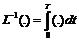Equation (2) can be hence as;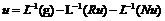(3)

The decomposition method represents the solution of equation (3) as the following infinite series: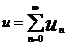(4)

The nonlinear operator Nu = Ψ(u) is decomposed as: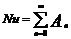(5)

where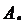are Adomian’s polynomials, which are defined as :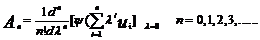(6)

Substituting equations (4) and (5) into equation (3), we have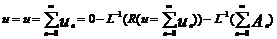(7)

Consequently, it can be written as: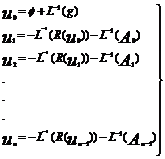(8)

where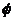is the initial condition,

Hence all the terms of u are calculated and the general solution obtained according to ADM as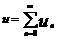. The convergent of this series has been proved in .

However, for some problems  this series can‘t be determined, so we use an approximation of the solution from truncated series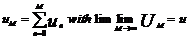(9)

1.2. Problem 1

Let us consider the Problem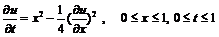(10)

ith the initial condition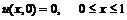(11)

Eq. (10) has the exact solution :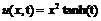(12)

In this problem we have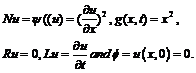By using Eq. (6), we obtain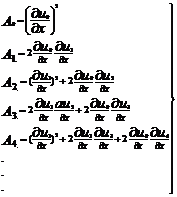(13)

By using Eq. (8), we have(14)

From Eq.(4) we have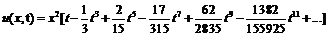(15)

which gives the exact solution (12).

1.3. Problem 2

Consider the nonlinear system of equations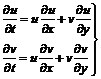(16)

with the initial conditions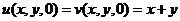(17)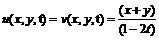(18)

In this problem Eqs. (16) can be written as: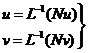(19)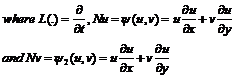By using Eq. (4) the solutions can be written as:(20)

The associated decomposition scheme is given by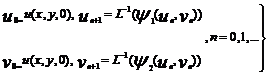(21)

We decompose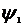and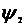according to the series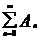and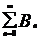respectively,

Whereand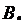are calculated by the Adomian’s polynomials which are defined in Eq.(6) then we obtain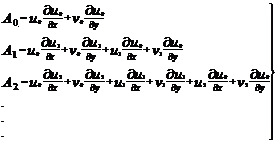(22)

Similarly: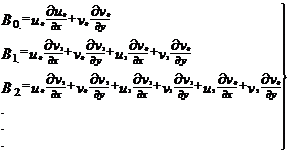(23)

By using Eq. (8) we have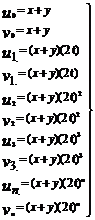(24)

From Eq.(4) we have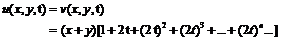(25)

which gives the exact solution (18).

The differential quadrature method (DQM) is a numerical technique used to solve the initial and boundary value problems. The DQM compared with the other numerical method such as the finite difference methods (FDM) and finite element methods (FEM) , and showing excellent numerical results , it needs only applying a few grid points in order to get high-precise solutions, a good convergence and it requires only less computational workload [13,14]. This method was proposed by Bellman in the early 70s [15,16]. Then, the technique has been successful employed in a variety of problems in engineering and physical sciences hence attracted many researchers attention in recent years. Al-Saif and Zhu , using the differential quadrature method to solve the coupled incompressible Navier–Stokes equation and heat equation and showing that accurate numerical results can be obtained by the DQM using only a few grid point and requires less storage and computational effort compared to the conventional low-order finite difference method. In another work, Al-saif and Zhu , using the mixed differential quadrature method(MDQM) for solving the coupled two-dimensional incompressible Navier - Stokes equation and heat equation. The results show that the new method is more accurate and has better convergence than the traditional DQM. The purpose of this paper is to introduce and application the differential quadrature method to solving unsteady state two-dimensional convection-diffusion equation .The results demonstrated that high accurate numerical solution by using only a few grid points and requires less storage and computational effort compared to the some numerical methods wealthy from some researchers in the precedent studies.

2. Discussion

In summary, the ADM is a powerful and e±cient technique for the solution of nonlinear ordinary, partial and fractional diferential equations. It provides the analyst with an easily computable, readily veriable and rapidly convergent sequence of analytic approximate functions for the solution.

Table 1. Comparison of ADM and DQM solutions for problem 1.

 ADM DQM t X 0.119 1.3854e-014 0 3.6576e-011 0.5 2.363e-013 0 1.6578e-010 0.1 0.789 6.4354e-013 0 2.7687e-010 0.5 0.119 6.5434e-006 6.5643e-013 8.6754e-009 0.5 8.6578e-007 1.4578e-013 6.1237e-009 0.789 4.45464e-005 2.2343e-013 9.5456e-008 1 0.119 8.3452-004 1.7656e-013 9.6754e-006 0.5 1.5464-003 1.4532e-013 3.4565e-006 0.789 6.4536-003 4.4565e-013 6.7876e-006

Table 2. Comparison of ADM and DQM solutions for problem 2

 ADM DQM t y X 0.125 0.125 1.0000e-004 4.3000e-006 3.8784e-007 0.784 3.0000e-004 3.3700e-005 1.1345e-006 0.5 0.5 3.0000e-004 3.3700e-005 1.1345e-006 0.1 0.125 0.125 3.0000e-004 3.3700e-005 1.1345e-006 0.784 6.0000e-004 3.3500e-005 1.7541e-006 0.2 0.125 0.125 4.4534e-003 5.5436e-003 2.6000e-005 0.784 1.6545e-003 1.4345e-005 1.0000e-004 0.5 0.5 1.6545e-003 1.3234e-005 1.0000e-004 0.125 0.125 1.6545e-003 1.2324e-005 1.0000e-004 0.784 2.4354e-003 3.3700e-005 1.7500e-004 0.3 0.125 0.125 4.4543e-003 3.4567e-003 1.7685e-004 0.784 1.7362e-002 3.3700e-005 6.4000e-004 0.5 0.5 1.7362e-002 1.5457e-003 6.4000e-004 0.125 0.125 1.7362e-002 1.4534e-003 6.4000e-004 0.784 2.3432e-002 2.3432e-002 1.4569e-003

3. Conclusion

In this paper, we have applied the Adomian decomposition method for solving three problems of nonlinear partial equations. We demonstrated that the decomposition procedure is quite efficient to determine the exact solutions. However, the method gives a simple powerful tool for obtaining the solutions without a need for large size of computations. It is also worth noting that the advantage of this method sometimes displays a fast convergence of the solutions. In addition, the numerical results which obtained by this method indicate a high degree of accuracy. The purpose of this paper is to introduce and application the differential quadrature method to solving unsteady state two-dimensional convection-diffusion equation .The results demonstrated that high accurate numerical solution by using only a few grid points and requires less storage and computational effort compared to the some numerical methods wealthy from some researchers in the precedent studies.

References

1. Al-saif A.S.J., (2007), "Numerical study for convection motion stability of thencompressibletwo-dimensional fluid flow by differential quadrature method", J.Basrah Researches(Sciences), Vol.33, No. 1. p.103-110.
2. Leveque R.J., (2006), "Finite difference methods for differential equations" A Math. 585. winters Quarter, University of Washington version of January.
3. Celik E., Bayram M. Yeloglu T., (2006), "Solution of differential–algebra EquationsbyAdomian decomposition method", Inter. J. Pure and Appl. Math. Sciences, Vol.3,No.1, p.93-100.
4. Javidi M. and Golbabai A., (2007), "Adomian decomposition method forapproximating thesolution of parabolic equations", J. Appl. Math. Sciences, Vol.1, No.5, p. 219-225.
5. S.E. Serrano (2011). Engineering Uncertainty and Risk Analysis: A Balanced Approach to Probability, Statistics, Stochas-btic Modeling, and Stochastic Diferential Equations, Second Revised Edition, HydroScience, Ambler, PA.
6. P.Y. Tsai and C.K. Chen (2010). An approximate analytic solution of the nonlinear Riccati diferential equation, J. Frank. Inst. 347 1850-1862.
7. A.M. Wazwaz (2011). Linear and Nonlinear Integral Equations: Methods and Applications, Higher Education Press, Beijing, and Springer-Verlag, Berlin.
8. J.S. Duan and R. Rach (2011). A new modi¯cation of the Adomian decomposition method for solving boundary value problems for higher order nonlinear diferential equations, Appl. Math. Comput. 218 ,4090-4118.
9. A.M. Wazwaz and R. Rach, (2011) . Comparison of the Adomian decomposition method and the variational iteration method for solving the Lane-Emden equations of the ¯rst and second kinds, Kybernetes 40 ,1305-1318.
10. A.M. Wazwaz (2012) . A reliable study for extensions of the Bratu problem with boundary conditions, Math. Methods Appl. Sci. 35 ,845-856.
11. Seng V. Abbaoui K. Cherruault Y., (1996), "Adomian’s polynomial for nonlinearoperators", J.Math. Comput. Modeling, Vol. 24, No. 1, p.59-65.
12. Bellman R., Kashef B.G. and Casti J., (1972), "Differential quadrature: A techniquefor therapid solution of nonlinear partial differential equations", J.comput. Phys.,Vol.10,No.1, p. 40-52.
13. H. Hossainzadeh, G. A. Afrouziand A. Yazdani (2011). Application of Adomian Decomposition Method for Solving ImpulsiveDifferential Equations.The Journal of Mathematics and Computer Science Vol .2 No.4 ,672-681.
14. Bert C. W. and Malik M .(2014)." Differential quadrature method in computational mechanics ",A review : Appl. Mech. Rev., 49, 1-27.
15. Shu C. , Chen W. and Du H.(2014)." Free vibration analysis of curvilinear quadrilateral platesby the DQ method", J. comput. Phys., 163, 452–466.
16. Al–Saif, A.S.J. and Zhu, Z.Y (2002).'' Differential quadrature method for solving the coupledincompressible Navier-Stockes equations and heat equation", Proc. 4th Int. Confer.on Nonlinear Mech., Shanghai, 897-901.
17. Ali A . N . H (2004). " Finite element methods to solve two-dimensional transport equation usingmodified Galerkin schemes ", M .Sc . thesis, Basrah University , Iraq.
18. Al-Saif,A. S. J. and Zhu Z.Y. (2003)." Application of Mixed Differential quadraturemethod forsolving the coupled tow-dimensional incompressible Navier–Stockes equation and heatequation ", J. of Shanghai University,7(4), 343 -351.

Article ToolsAbstractPDF(202K)